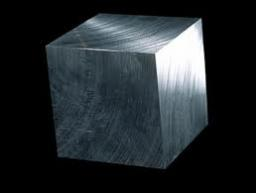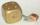Calculate the edge of the cube made ​​from lead, which weighs 19 kg. The density of lead is 11341 kg/m3.

a =  11.9 cm

### Step-by-step explanation:Did you find an error or inaccuracy? Feel free to write us. Thank you!Tips to related online calculators
Tip: Our volume units converter will help you with the conversion of volume units.
Tip: Our Density units converter will help you with the conversion of density units.

## Related math problems and questions:

• SeawaterSeawater has a density of 1025 kg/m3, ice 920 kg/m3. 8 liters of seawater froze and created a cube. Calculate the size of the cube edge.
• CubeThe cube weighs 11 kg. How weight is a cube of the same material if its dimensions are 3-times smaller?
• Eiffel TowerEiffel Tower in Paris is 300 meters high and is made of steel. Its weight is 8000 tons. How tall is the tower model made ​​of the same material if it weighs is 2.4 kg?
• Iron sphereIron sphere has weight 100 kg and density ρ = 7600 kg/m3. Calculate the volume, surface, and diameter of the sphere.
• CuZnBrass is an alloy of copper and zinc. The 10 centimeter brass cube has a weight of 8.6 kg. Copper density is 8930 kg/m3, the zinc density is 7130 kg/m3. Calculate how many kg of copper and zinc a cube contains.
• Solid in waterThe solid weighs in air 16.1 kg and in water 13 kg. Calculate the density of the solid.
• Hollow sphereThe steel hollow sphere floats on the water plunged into half its volume. Determine the outer radius of the sphere and wall thickness, if you know that the weight of the sphere is 0.5 kg and the density of steel is 7850 kg/m3
• Cone from cubeFrom a wooden block, 20 cm high was the turned largest possible cone. Calculate its weight if you know that the density of wood was 850 kg/m3
• Steel tubeThe steel tube has an inner diameter of 4 cm and an outer diameter of 4.8 cm. The density of the steel is 7800 kg/m3. Calculate its length if it weighs 15 kg.
• Cuboid 5Calculate the mass of the cuboid with dimensions of 12 cm; 0.8 dm and 100 mm made from spruce wood (density = 550 kg/m3).
• BucketThe bucket half filled with water weighs 5.55 kg, the full bucket weighs 9.85 kg. How much does the bucket weigh?
• Copper sheetThe copper plate has a length of 1 m, width 94 cm and weighs 9 kg. What is the plate thickness, if 1 m3 weighs 8715 kg?
• Reservoir + waterThe reservoir filled with water weighs 12 kg and after pouring off three-quarters of the water weights 3 kg. Calculate the weight and volume of the reservoir.
• The prison ballCalculate the density of the material that the prison ball is made from if you know its diameter is 15cm and its weight is approximately 2.3kg. With the help of mathematical-physicochemical tables estimate what material the ball is made from.
• SphereThe surface of the sphere is 12100 cm2, and the weight is 136 kg. What is its density?
• SnowSnow fell overnight layer of thickness 19 cm. In the morning I had to clear a path 69 m long and one meter wide. How many cubic meters of snow I clear? How many kilos was it? (1 m3 fresh snow weighs 350 kg)
• Wall thicknessThe hollow metal ball has an outside diameter of 40 cm. Determine the wall thickness if the weight is 25 kg and the metal density is 8.45 g/cm3.